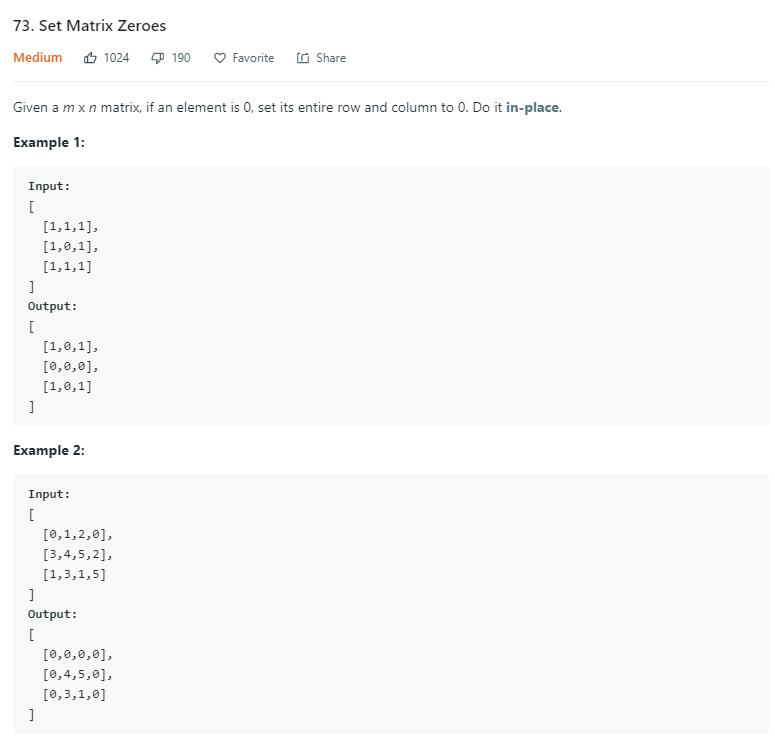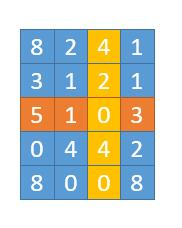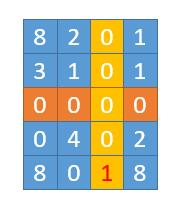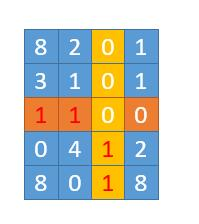# 题目描述（中等难度）# 解法一

public void setZeroes(int[][] matrix) {
int row = matrix.length;
int col = matrix.length;
int[][] matrix_copy = new int[row][col];
//复制矩阵
for (int i = 0; i < row; i++) {
for (int j = 0; j < col; j++) {
matrix_copy[i][j] = matrix[i][j];
}
}
for (int i = 0; i < row; i++) {
for (int j = 0; j < col; j++) {
//找到 0 的位置
if (matrix_copy[i][j] == 0) {
//将当前行，当前列置为 0
setRowZeroes(matrix, i);
setColZeroes(matrix, j);
}

}
}
}
//第 col 列全部置为 0
private void setColZeroes(int[][] matrix, int col) {
for (int i = 0; i < matrix.length; i++) {
matrix[i][col] = 0;
}
}
//第 rol 行全部置为 0
private void setRowZeroes(int[][] matrix, int row) {
for (int i = 0; i < matrix[row].length; i++) {
matrix[row][i] = 0;
}
}


# 解法二

public void setZeroes(int[][] matrix) {
int row = matrix.length;
int col = matrix.length;
//用两个 bool 数组标记当前行和当前列是否需要置为 0
boolean[] row_zero = new boolean[row];
boolean[] col_zero = new boolean[col];
for (int i = 0; i < row; i++) {
for (int j = 0; j < col; j++) {
//找到 0 的位置
if (matrix[i][j] == 0) {
row_zero[i] = true;
col_zero[j] = true;
}
}
}
//将行标记为 true 的行全部置为 0
for (int i = 0; i < row; i++) {
if (row_zero[i]) {
setRowZeroes(matrix, i);
}
}
//将列标记为 false 的列全部置为 0
for (int i = 0; i < col; i++) {
if (col_zero[i]) {
setColZeroes(matrix, i);
}
}
}
//第 col 列全部置为 0
private void setColZeroes(int[][] matrix, int col) {
for (int i = 0; i < matrix.length; i++) {
matrix[i][col] = 0;
}
}
//第 rol 行全部置为 0
private void setRowZeroes(int[][] matrix, int row) {
for (int i = 0; i < matrix[row].length; i++) {
matrix[row][i] = 0;
}
}


class Solution {
public void setZeroes(int[][] matrix) {
int R = matrix.length;
int C = matrix.length;
Set<Integer> rows = new HashSet<Integer>();
Set<Integer> cols = new HashSet<Integer>();

// 将元素为 0 的地方的行和列存起来
for (int i = 0; i < R; i++) {
for (int j = 0; j < C; j++) {
if (matrix[i][j] == 0) {
}
}
}

//将存储的 Set 拿出来，然后将当前行和列相应的元素置零
for (int i = 0; i < R; i++) {
for (int j = 0; j < C; j++) {
if (rows.contains(i) || cols.contains(j)) {
matrix[i][j] = 0;
}
}
}
}
}


# 解法三

47题解法二 的思路，就是假设我们对问题足够的了解，假设存在一个数，矩阵中永远不会存在，然后我们就可以把需要变成 0 的位置先变成这个数，也就是先标记一下，最后再统一把这个数变成 0。直接贴下leetcode解法二的代码。

class Solution {
public void setZeroes(int[][] matrix) {
int MODIFIED = -1000000; //假设这个数字不存在于矩阵中
int R = matrix.length;
int C = matrix.length;

for (int r = 0; r < R; r++) {
for (int c = 0; c < C; c++) {
//找到等于 0 的位置
if (matrix[r][c] == 0) {
// 将需要变成 0 的行和列改为之前定义的数字
// 如果是 0 不要管，因为我们要找 0 的位置
for (int k = 0; k < C; k++) {
if (matrix[r][k] != 0) {
matrix[r][k] = MODIFIED;
}
}
for (int k = 0; k < R; k++) {
if (matrix[k][c] != 0) {
matrix[k][c] = MODIFIED;
}
}
}
}
}

for (int r = 0; r < R; r++) {
for (int c = 0; c < C; c++) {
// 将是定义的数字的位置变成 0
if (matrix[r][c] == MODIFIED) {
matrix[r][c] = 0;
}
}
}
}
}public void setZeroes(int[][] matrix) {
int row = matrix.length;
int col = matrix.length;
int free_row = -1; //记录第一个 0 出现的行
int free_col = -1; //记录第一个 0 出现的列
for (int i = 0; i < row; i++) {
for (int j = 0; j < col; j++) {
//如果是当前作为标记的列，就跳过
if (j == free_col) {
continue;
}
if (matrix[i][j] == 0) {
//判断是否是第一个 0
if (free_row == -1) {
free_row = i;
free_col = j;
//初始化行标记位为 0，如果之前是 0 就置为 1
for (int k = 0; k < matrix.length; k++) {
if (matrix[k][free_col] == 0) {
matrix[k][free_col] = 1;
} else {
matrix[k][free_col] = 0;
}

}
//初始化列标记位为 0，如果之前是 0 就置为 1
for (int k = 0; k < matrix[free_row].length; k++) {
if (matrix[free_row][k] == 0) {
matrix[free_row][k] = 1;
} else {
matrix[free_row][k] = 0;
}
}
break;
//找 0 的位置，将相应的标志置 1
} else {
matrix[i][free_col] = 1;
matrix[free_row][j] = 1;
}
}

}
}
if (free_row != -1) {
//将标志位为 1 的所有列置为 0
for (int i = 0; i < col; i++) {
if (matrix[free_row][i] == 1) {
setColZeroes(matrix, i);
}
}
//将标志位为 1 的所有行置为 0
for (int i = 0; i < row; i++) {
if (matrix[i][free_col] == 1) {
setRowZeroes(matrix, i);
}
}
}
}

private void setColZeroes(int[][] matrix, int col) {
for (int i = 0; i < matrix.length; i++) {
matrix[i][col] = 0;
}
}

private void setRowZeroes(int[][] matrix, int row) {
for (int i = 0; i < matrix[row].length; i++) {
matrix[row][i] = 0;
}
}


leetcode解法三和我的思想是一样的，它标记位直接用第一行和第一列，由于第一行和第一列不一定会被置为 0，所以需要用 isCol 变量来标记第一列是否需要置为 0，用 matrix 标记第一行是否需要置为 0。它是将用 0 表示当前行（列）需要置 0，这一点也很巧妙，相比我上边的算法就不需要初始化标记位了。

class Solution {
public void setZeroes(int[][] matrix) {
Boolean isCol = false;
int R = matrix.length;
int C = matrix.length;
for (int i = 0; i < R; i++) {
//判断第 1 列是否需要置为 0
if (matrix[i] == 0) {
isCol = true;
}
//找 0 的位置，将相应标记置 0
for (int j = 1; j < C; j++) {
if (matrix[i][j] == 0) {
matrix[j] = 0;
matrix[i] = 0;
}
}
}
//根据标志，将元素置 0
for (int i = 1; i < R; i++) {
for (int j = 1; j < C; j++) {
if (matrix[i] == 0 || matrix[j] == 0) {
matrix[i][j] = 0;
}
}
}

//判断第一行是否需要置 0
if (matrix == 0) {
for (int j = 0; j < C; j++) {
matrix[j] = 0;
}
}

//判断第一列是否需要置 0
if (isCol) {
for (int i = 0; i < R; i++) {
matrix[i] = 0;
}
}
}
}